International Tables for Crystallography (2006). Vol. C. ch. 4.2, pp. 191-258https://doi.org/10.1107/97809553602060000592

## Contents

• 4.2. X-rays  (pp. 191-258)
• 4.2.1. Generation of X-rays  (pp. 191-200) | html | pdf |
• 4.2.1.1. The characteristic line spectrum  (pp. 191-192) | html | pdf |
• 4.2.1.1.1. The intensity of characteristic lines  (pp. 191-192) | html | pdf |
• 4.2.1.2. The continuous spectrum  (pp. 192-193) | html | pdf |
• 4.2.1.3. X-ray tubes  (pp. 193-195) | html | pdf |
• 4.2.1.3.1. Power dissipation in the anode  (p. 195) | html | pdf |
• 4.2.1.4. Radioactive X-ray sources  (pp. 195-196) | html | pdf |
• 4.2.1.5. Synchrotron-radiation sources  (pp. 196-198) | html | pdf |
• 4.2.1.6. Plasma X-ray sources  (pp. 198-199) | html | pdf |
• 4.2.1.7. Other sources of X-rays  (pp. 199-200) | html | pdf |
• 4.2.2. X-ray wavelengths  (pp. 200-212) | html | pdf |
• 4.2.2.1. Historical introduction  (pp. 200-201) | html | pdf |
• 4.2.2.2. Known problems  (p. 201) | html | pdf |
• 4.2.2.3. Alternative strategies  (p. 201) | html | pdf |
• 4.2.2.4. The X-ray wavelength scales, old and new  (pp. 201-202) | html | pdf |
• 4.2.2.5. K -series reference wavelengths  (p. 202) | html | pdf |
• 4.2.2.6. L -series reference wavelengths  (p. 202) | html | pdf |
• 4.2.2.7. Absorption-edge locations  (pp. 202-204) | html | pdf |
• 4.2.2.8. Outline of the theoretical procedures  (pp. 204-205) | html | pdf |
• 4.2.2.9. Evaluation of the uncorrelated energy with the Dirac–Fock method  (p. 205) | html | pdf |
• 4.2.2.10. Correlation and Auger shifts  (p. 205) | html | pdf |
• 4.2.2.11. QED corrections  (pp. 205-208) | html | pdf |
• 4.2.2.12. Structure and format of the summary tables  (pp. 211-212) | html | pdf |
• 4.2.2.13. Availability of a more complete X-ray wavelength table  (p. 212) | html | pdf |
• 4.2.2.14. Connection with scales used in previous literature  (p. 212) | html | pdf |
• 4.2.3. X-ray absorption spectra  (pp. 213-220) | html | pdf |
• 4.2.3.1. Introduction  (pp. 213-214) | html | pdf |
• 4.2.3.1.1. Definitions  (p. 213) | html | pdf |
• 4.2.3.1.2. Variation of X-ray attenuation coefficients with photon energy  (p. 213) | html | pdf |
• 4.2.3.1.3. Normal attenuation, XAFS, and XANES  (pp. 213-214) | html | pdf |
• 4.2.3.2. Techniques for the measurement of X-ray attenuation coefficients  (pp. 214-215) | html | pdf |
• 4.2.3.2.1. Experimental configurations  (pp. 214-215) | html | pdf |
• 4.2.3.2.2. Specimen selection  (p. 215) | html | pdf |
• 4.2.3.2.3. Requirements for the absolute measurement of μ l or (μ/ρ)  (p. 215) | html | pdf |
• 4.2.3.3. Normal attenuation coefficients  (p. 215) | html | pdf |
• 4.2.3.4. Attenuation coefficients in the neighbourhood of an absorption edge  (pp. 216-219) | html | pdf |
• 4.2.3.4.1. XAFS  (pp. 216-219) | html | pdf |
• 4.2.3.4.1.1. Theory  (pp. 216-217) | html | pdf |
• 4.2.3.4.1.2. Techniques of data analysis  (pp. 217-218) | html | pdf |
• 4.2.3.4.1.3. XAFS experiments  (pp. 218-219) | html | pdf |
• 4.2.3.4.2. X-ray absorption near edge structure (XANES)  (p. 219) | html | pdf |
• 4.2.3.5. Comments  (p. 220) | html | pdf |
• 4.2.4. X-ray absorption (or attenuation) coefficients  (pp. 220-229) | html | pdf |
• 4.2.4.1. Introduction  (pp. 220-221) | html | pdf |
• 4.2.4.2. Sources of information  (pp. 221-229) | html | pdf |
• 4.2.4.2.1. Theoretical photo-effect data: σ pe   (p. 221) | html | pdf |
• 4.2.4.2.2. Theoretical Rayleigh scattering data: σ R   (pp. 221-229) | html | pdf |
• 4.2.4.2.3. Theoretical Compton scattering data: σ C   (p. 229) | html | pdf |
• 4.2.4.3. Comparison between theoretical and experimental data sets  (p. 229) | html | pdf |
• 4.2.4.4. Uncertainty in the data tables  (p. 229) | html | pdf |
• 4.2.5. Filters and monochromators  (pp. 229-241) | html | pdf |
• 4.2.5.1. Introduction  (pp. 229-236) | html | pdf |
• 4.2.5.2. Mirrors and capillaries  (pp. 236-238) | html | pdf |
• 4.2.5.2.1. Mirrors  (pp. 236-237) | html | pdf |
• 4.2.5.2.2. Capillaries  (p. 237) | html | pdf |
• 4.2.5.2.3. Quasi-Bragg reflectors  (pp. 237-238) | html | pdf |
• 4.2.5.3. Filters  (p. 238) | html | pdf |
• 4.2.5.4. Monochromators  (pp. 238-241) | html | pdf |
• 4.2.5.4.1. Crystal monochromators  (pp. 238-239) | html | pdf |
• 4.2.5.4.2. Laboratory monochromator systems  (p. 239) | html | pdf |
• 4.2.5.4.3. Multiple-reflection monochromators for use with laboratory and synchrotron-radiation sources  (pp. 239-240) | html | pdf |
• 4.2.5.4.4. Polarization  (pp. 240-241) | html | pdf |
• 4.2.6. X-ray dispersion corrections  (pp. 241-258) | html | pdf |
• 4.2.6.1. Definitions  (pp. 242-243) | html | pdf |
• 4.2.6.1.1. Rayleigh scattering  (p. 242) | html | pdf |
• 4.2.6.1.2. Thomson scattering by a free electron  (p. 242) | html | pdf |
• 4.2.6.1.3. Elastic scattering from electrons bound to atoms: the atomic scattering factor, the atomic form factor, and the dispersion corrections  (pp. 242-243) | html | pdf |
• 4.2.6.2. Theoretical approaches for the calculation of the dispersion corrections  (pp. 243-248) | html | pdf |
• 4.2.6.2.1. The classical approach  (pp. 243-244) | html | pdf |
• 4.2.6.2.2. Non-relativistic theories  (pp. 244-245) | html | pdf |
• 4.2.6.2.3. Relativistic theories  (pp. 245-248) | html | pdf |
• 4.2.6.2.3.1. Cromer and Liberman: relativistic dipole approach   (pp. 245-246) | html | pdf |
• 4.2.6.2.3.2. The scattering matrix formalism  (pp. 246-248) | html | pdf |
• 4.2.6.2.4. Intercomparison of theories  (p. 248) | html | pdf |
• 4.2.6.3. Modern experimental techniques  (pp. 248-258) | html | pdf |
• 4.2.6.3.1. Determination of the real part of the dispersion correction: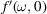(pp. 248-250) | html | pdf |
• 4.2.6.3.2. Determination of the real part of the dispersion correction: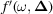(pp. 250-251) | html | pdf |
• 4.2.6.3.2.1. Measurements using the dynamical theory of X-ray diffraction  (pp. 250-251) | html | pdf |
• 4.2.6.3.2.2. Friedel- and Bijvoet-pair techniques  (p. 251) | html | pdf |
• 4.2.6.3.3. Comparison of theory with experiment  (pp. 251-258) | html | pdf |
• 4.2.6.3.3.1. Measurements in the high-energy limit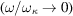(pp. 251-252) | html | pdf |
• 4.2.6.3.3.2. Measurements in the vicinity of an absorption edge  (pp. 252-253) | html | pdf |
• 4.2.6.3.3.3. Accuracy in the tables of dispersion corrections  (p. 253) | html | pdf |
• 4.2.6.3.3.4. Towards a tensor formalism  (pp. 253-258) | html | pdf |
• 4.2.6.3.3.5. Summary  (p. 258) | html | pdf |
• 4.2.6.4. Table of wavelengths, energies, and linewidths used in compiling the tables of the dispersion corrections  (p. 258) | html | pdf |
• 4.2.6.5. Tables of the dispersion corrections for forward scattering, averaged polarization using the relativistic multipole approach  (p. 258) | html | pdf |
• References | html | pdf |
• Figures
• Fig. 4.2.1.1. f (χ) curves for Cu K-L 3 at a series of different accelerating voltages (in kV)  (p. 192) | html | pdf |
• Fig. 4.2.1.2. Experimental measurements of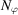for Cu K-L 3 as functions of the accelerating voltage for different take-off angles  (p. 193) | html | pdf |
• Fig. 4.2.1.3. Intensity per unit frequency interval versus frequency in the continuous spectrum from a thick target at different accelerating voltages  (p. 193) | html | pdf |
• Fig. 4.2.1.4. The function μ in Müller's equation (equation 4.2.1.12) as a function of the ratio of width to length of the focal spot  (p. 195) | html | pdf |
• Fig. 4.2.1.5. Synchrotron radiation emitted by a relativistic electron travelling in a curved trajectory  (p. 197) | html | pdf |
• Fig. 4.2.1.6. Synchrotron-radiation spectrum: percentage per unit wavelength interval ( a ) of power of total power and ( b ) of number of photons of total number of photons at wavelengths greater than λ versus λ/λ c   (p. 197) | html | pdf |
• Fig. 4.2.1.7. Main components of a dedicated electron storage-ring synchrotron-radiation source  (p. 198) | html | pdf |
• Fig. 4.2.1.8. Electron trajectory within a multipole wiggler or undulator  (p. 198) | html | pdf |
• Fig. 4.2.1.9. Spectral distribution and critical wavelengths for ( a ) a dipole magnet, ( b ) a wavelength shifter, and ( c ) a multipole wiggler for the proposed ESRF  (p. 198) | html | pdf |
• Fig. 4.2.1.10. Comparison of the spectra from the storage ring SPEAR in photons s −1  mA −1  mrad −1  per 1% passband (1978 performance) and a rotating-anode X-ray generator  (p. 198) | html | pdf |
• Fig. 4.2.1.11. The evolution of storage-ring synchrotron-radiation sources over the decades, as illustrated by their increasing number and range of machine energies (based on Suller, 1992)  (p. 200) | html | pdf |
• Fig. 4.2.1.12. X-ray emission from various laser-produced plasmas  (p. 200) | html | pdf |
• Fig. 4.2.2.1. Relative deviations between theoretical and experimental results for K -series spectra  (p. 212) | html | pdf |
• Fig. 4.2.2.2. Comparison of L -series data with experiment for the indicated range of Z   (p. 212) | html | pdf |
• Fig. 4.2.3.1. Theoretical cross sections for photon interactions with carbon showing the contributions of photoelectric, elastic (Rayleigh), inelastic (Compton), and pair-production cross sections to the total cross sections  (p. 213) | html | pdf |
• Fig. 4.2.3.2. The dependence of the X-ray attenuation coefficient on energy for a range of germanium compounds, taken in the neighbourhood of the germanium absorption edge (from IT IV, 1974)  (p. 214) | html | pdf |
• Fig. 4.2.3.3. Schematic representations of experimental apparatus used in the IUCr X-ray Attenuation Project (Creagh & Hubbell, 1987; Creagh, 1985)  (p. 214) | html | pdf |
• Fig. 4.2.3.4. Steps in the reduction of data from an XAFS experiment using the Fourier transform technique: ( a ) after the removal of background χ( k ) versus k ; ( b ) after multiplication by a weighting function (in this case k 3 ); ( c ) after Fourier transformation to determine r ′  (p. 217) | html | pdf |
• Fig. 4.2.3.5. Schematic representations of the scattering processes undergone by the ejected photoelectron in the single-scattering (XAFS) case and the full multiple-scattering regime (XANES)  (p. 219) | html | pdf |
• Fig. 4.2.4.1. Agreement between theory and experiment for oxygen ( Z = 8) in the `soft' X-ray region  (p. 220) | html | pdf |
• Fig. 4.2.4.2. The total cross section for silicon ( Z = 14) compared with the unrenormalized Scofield values  (p. 221) | html | pdf |
• Fig. 4.2.4.3. The total cross section for uranium ( Z = 92): The theoretical values (solid line) are partially obscured by the high density of available measurements  (p. 222) | html | pdf |
• Fig. 4.2.4.4. Comparison between this tabulation and experimental data contained in Saloman & Hubbell (1986)  (p. 222) | html | pdf |
• Fig. 4.2.5.1. The variation of specular reflectivity with incident photon energy is shown for materials of different atomic number and a constant angle of incidence of 0.2°  (p. 236) | html | pdf |
• Fig. 4.2.5.2. The use of mirrors in a typical synchrotron-radiation beamline  (p. 237) | html | pdf |
• Fig. 4.2.5.3. The reflectivity of a multiple-quantum-well device is shown  (p. 238) | html | pdf |
• Fig. 4.2.5.4. In ( a ), the schematic rocking curve for a silicon crystal in the neighbourhood of the 111 Bragg peak is shown  (p. 239) | html | pdf |
• Fig. 4.2.5.5. A schematic diagram of a beamline designed to produce circularly polarized light from initially linearly polarized light using Laue-case reflections  (p. 240) | html | pdf |
• Fig. 4.2.5.6. A schematic diagram of a Hart-type tuneable channel-cut monochromator is shown  (p. 241) | html | pdf |
• Fig. 4.2.5.7. A schematic diagram of the use of a Bragg–Fresnel lens to focus hard X-rays onto a high-pressure cell  (p. 241) | html | pdf |
• Fig. 4.2.6.1. The relativistic correction in electrons per atom for: ( a ) the modified form-factor approach; ( b ) the relativistic multipole approach; ( c ) the relativistic dipole approach  (p. 247) | html | pdf |
• Fig. 4.2.6.2. Measured values of f ′(ω, 0) at the K -edge of Nb in LiNbO 3 and the Kramers–Kronig transformation of f ′′(ω, 0)  (p. 247) | html | pdf |
• Tables
• Table 4.2.1.1. Correspondence between X-ray diagram levels and electron configurations  (p. 191) | html | pdf |
• Table 4.2.1.2. Correspondence between IUPAC and Siegbahn notations for X-ray diagram lines  (p. 191) | html | pdf |
• Table 4.2.1.3. Copper-target X-ray tubes and their loading  (p. 194) | html | pdf |
• Table 4.2.1.4. Relative permissible loading for different target materials  (p. 196) | html | pdf |
• Table 4.2.1.5. Radionuclides decaying wholly by electron capture, and yielding little or no γ-radiation  (p. 196) | html | pdf |
• Table 4.2.1.6. Comparison of storage-ring synchrotron-radiation sources  (p. 199) | html | pdf |
• Table 4.2.1.7. Intensity gain with storage rings over conventional sources  (p. 200) | html | pdf |
• Table 4.2.2.1. K -series reference wavelengths in Å  (p. 203) | html | pdf |
• Table 4.2.2.2. Directly measured L -series reference wavelengths in Å  (p. 204) | html | pdf |
• Table 4.2.2.3. Directly measured and emission + binding energies (see text) K -absorption edges in Å  (p. 205) | html | pdf |
• Table 4.2.2.4. Wavelengths of K -emission lines and K -absorption edges in Å  (pp. 206-208) | html | pdf |
• Table 4.2.2.5. Wavelengths of L -emission lines and L -absorption edges in Å  (pp. 209-211) | html | pdf |
• Table 4.2.2.6. Wavelength conversion factors  (p. 212) | html | pdf |
• Table 4.2.3.1. Some synchrotron-radiation facilities providing XAFS databases and analysis utilities  (p. 219) | html | pdf |
• Table 4.2.4.1. Table of wavelengths and energies for the characteristic radiations used in Tables 4.2.4.2 and 4.2.4.3  (p. 221) | html | pdf |
• Table 4.2.4.2. Total phonon interaction cross section  (pp. 223-229) | html | pdf |
• Table 4.2.4.3. Mass attenuation coefficients  (pp. 230-236) | html | pdf |
• Table 4.2.6.1. Values of E tot / mc 2 listed as a function of atomic number Z   (p. 246) | html | pdf |
• Table 4.2.6.2. Comparison between the S -matrix calculations of Kissel (K) (1977) and the form-factor calculations of Cromer & Liberman (C & L) (1970, 1981, 1983) and Creagh & McAuley (C & M) for the noble gases and several common metals  (p. 249) | html | pdf |
• Table 4.2.6.3. A comparison of the forward-scattering amplitudes computed using different theoretical approaches  (p. 250) | html | pdf |
• Table 4.2.6.4. Comparison of measurements of the real part of the dispersion correction for LiF, Si, Al and Ge for characteristic wavelengths Ag K α 1 , Mo K α 1 and Cu K α 1 with theoretical predictions  (p. 252) | html | pdf |
• Table 4.2.6.5. Comparison of measurements of f ′(ω, 0) for C, Si and Cu for characteristic wavelengths Ag K α 1 , Mo K α 1 and Cu K α 1 with theoretical predictions  (p. 253) | html | pdf |
• Table 4.2.6.6. Comparison of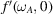for copper, nickel, zirconium, and niobium for theoretical and experimental data sets  (p. 254) | html | pdf |
• Table 4.2.6.7. Lists of wavelengths, energies, and linewidths used in compiling the table of dispersion corrections  (p. 254) | html | pdf |
• Table 4.2.6.8. Dispersion corrections for forward scattering  (pp. 255-257) | html | pdf |